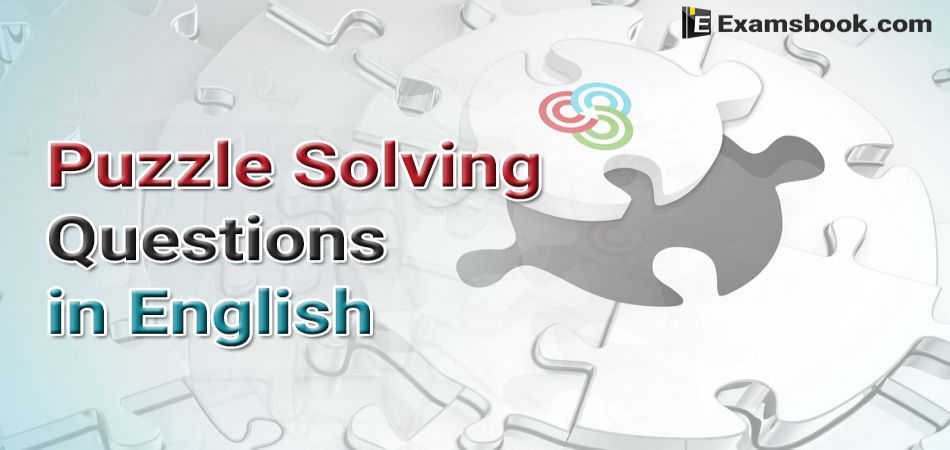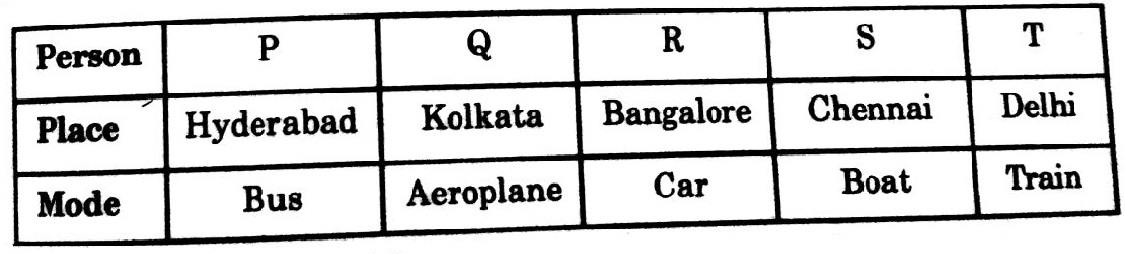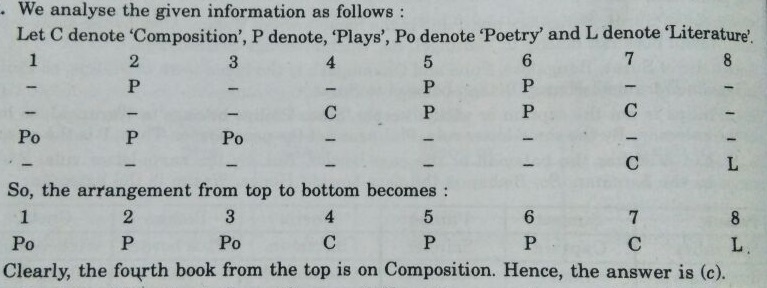• Save

# Puzzle Solving Questions with Answers in English

6 days ago 172 Views
NEWPuzzle questions ask in the exams, to check your mental ability. But some students are frequently Puzzled in puzzle solving questions in the competitive exams. Keeping in mind that, here I am sharing important puzzle questions with answers in English with its solutions from which students can understand this topic how to solve these type of questions.

To get a better result in competitive exams, you should try to solve these puzzle questions yourself. These questions will be helpful for your upcoming exams.

## Puzzle Questions and Answers with Solutions

Direction(1-5). Read the following information carefully and answer the questions that follow

(i) Five friends P, Q, R, S and T travelled to five different cities of Chennai, Kolkata, Delhi, Bangalore and Hyderabad by different modes of transport of Bus, Train, Aeroplane, Car and Boat from Mumbai.

(ii) The person who travelled to Delhi did not travel by boat.

(iii) R went to Bangalore by car and Q went to Kolkata by aeroplane.

(iv) S travelled by boat whereas T travelled by train.

(v) Mumbai is not connected by bus to Delhi and Chennai.

Q.1. Which of the following combinations of person and mode is not correct?

(A) P - Bus

(B) Q - Aeroplane

(C) R – Car

(D) S – Boat

(E) T – Aeroplane

Ans .  E

Q.2. Which of the following combinations is true for S?

(a) Delhi Bus

(b) Chennai Bus

(c) Chennai Boat

(e) None of these

Ans .  C

Q.3. Which of the following combinations of place and mode is not correct?

(a) Delhi Bus

(b) Kolkata - Aeroplane

(c) Bangalore - Car

(d) Chennai - Boat

(e) Hyderabad - Bus

Ans .  A

Q.4. The person travelling to Delhi went by which of the following modes?

(a) Bus

(b) Train

(c) Aeroplane

(d) Car

(e) Boat

Ans .  B

Q.5. Who among the following travelled to Delhi?

(a) R

(b) S

(c) T

(e) None of these

Ans .  C

Solution(1 to 5)

The given information can be analysed as follows:

(A) Mode of Transport: R travels by Car, Q by Aeroplane, S by Boat and T by Train. Now, only P remains. So, P travels by Bus.

(B) Place of Travel: R goes to Bangalore, Q to Kolkata. Now, bus transport is not available for Delhi or Chennai. So, P who travels by Bus goes to Hyderabad. S travels by boat and hence, by (ii), did not go to Delhi. So, S goes to Chennai. Now, only T remains. So, T goes to Delhi.1. Clearly, the incorrect combination is T – Aeroplane. So, the answer is (e).

2. Clearly, the correct combination for S is Chennai – Boat. So, the answer is (c).

3. Clearly, the incorrect combination is Delhi – Bus. So, the answer is (a).

4. Clearly, T travelled to Delhi by train. So, the answer is (b).

5. Clearly, T travelled to Delhi. The answer is (c).

Q.6. Eight books are kept one over the other. Counting from the top, the second, fifth and sixth books are on Plays. Two books on Plays are between two books on Composition. One book of Plays is between two books Poetry while the book at the top of the book of Literature is a book of Composition. Which book is fourth from the top?

(A) Plays

(B) Poetry

(C) composition

(D) Literature

Ans .  CDirection (7 to 9): Read the information given below to answer these question:

(i) Aarti is older than Sanya.

(ii) Muskan is elder than Aarti but younger than Kashish.

(iii) Kashish is elder than Sanya.

(iii) Sanya is younger than Muskan.

(iv) Gargi is the eldest.

Q.7. Who is the youngest?

(A) Kashish

(B) Aarti

(C) Muskan

(D) Sanya

Ans .  D

Q.8. Age wise, who is in the middle?

(A) Kashish

(B) Aarti

(C) Muskan

(D) Sanya

Ans .  C

Q.9. Which of the given statement is/are superfluous and can be dispensed with while answering the above questions?

(A) Either (i) or (iii)

(B) Only (iv)

(C) Either (i) or (iv)

(D) Both (iii) and (iv)

(E) None of these

Ans .  D

Solution(7 to 9)

Let the first letter of the name of each girl denote her age.

From (i), we have : A > S.

From (ii), we have : K > M > A.

From (iii), we have : K > S.

From (iv), we have : S < M.

Combining these, we get : K > M > A > S.

Since Gargi is the eldest, so we have : G > K > M > A > S.

7. (d): Sanya is the youngest.

8. (c): Age wise, Muskan is in the middle.

9. (d): From (i) and (ii), we have: K > M > A > S. This indicates both K > S and S < M which are given in (iii) and (iv). Thus, both (iii) and (iv) are superfluous.

Direction (10 - 11): These questions are based on the following information :

Five men A, B, C, D and E read a newspaper. The one who reads first gives it to C. The one who reads last had taken from A. E was not the first or last to read. There were two readers between B and A.

Q.10. B passed the newspaper to whom?

(A) A

(B) C

(C) D

(D) E

(E) None of these

Ans .  B

Q.11. Who read the newspaper last?

(A) A

(B) B

(C) C

(D) D

(E) None of these.

Ans .  D

Solution(10 to 11)

C is the second reader. A is the second last reader.

E is not the first or last to read. So, E is the third reader. There were two readers between B and A.

So, the order of reading the newspaper is: B, C, E, A, D.

10. (b): B passed the newspaper to C.

11. (d): D read the newspaper last.